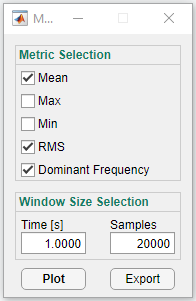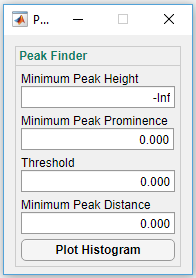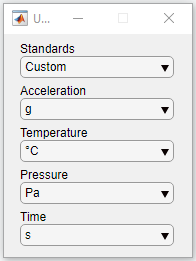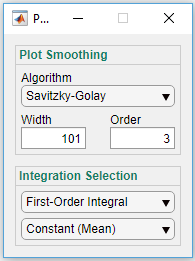# Analyzer Utilities

These utilities can be accessed under the tools menu item in the Analyzer.  These are helpful at quickly summarizing a larger file and for finding areas of interest in the file.

# Video Walk through

Video Walk through Below is a quick video which walks through the conversion process.# Moving Metrics

Moving metrics (also known as rolling metrics), calculate an array of local k-point metric values, where each metric is calculated over a sliding window of length k across neighboring elements.  Moving metrics are generally helpful as they reduce the size of your data and help transform it to a more meaningful measurement.  One example of this is using the RMS of an acceleration signal to estimate the total energy of a system.

The following metrics are supported by the Analyzer:

• Mean: uses the arithmetic mean (average)
• Max: uses the maximum value
• Min: uses the minimum value
• RMS: uses the root mean square metric, which is the square root of the arithmetic mean of the squares of the given values
• Dominant Frequency:  calculates the FFT of the window and plots both the highest amplitude in that signal and the magnitude of that frequency bin.

All moving metrics are calculated with no overlap between windows.To use the moving metrics tool in the Analyzer, first select the data you’d like to view as you normally would in the channel selector window.  Then in the moving metrics window (seen above), select all the metrics you’d like to produce graphs for, and your desired window size (The length of the sliding window to be used).  Once these have been specified, pressing the ‘Plot’ button will produce a graph for each of the metrics selected, and pressing the “Export” button will produce a .csv containing all the moving metric data.

# Peak Finding

The peak finding tool is used to generate a histogram of the local maxima (peaks) in data.  This can be used in a wide range of circumstances, for example it can be used to count the number of shock events which have a specific amplitude on a large file.

You are given 4 parameters to specify what is considered a peak:

• Minimum Peak Height: The minimum height for something to be considered a ‘peak’
• Minimum Peak Prominence: The minimum prominence for a peak
• Threshold: Minimum height difference between a peak and its neighbors
• Minimum Peak Distance: Minimum horizontal distance between neighboring peaks

(for reproducibility, these four parameters are fed into MATLAB’s findpeaks function, and all other parameters use the default values)# Unit Selection

Having inconsistent units within the same project is at best an inconvenience.  With this in mind, the Analyzer has a unit selection tool (‘unit selector’), which allows you to specify the units desired for each of the measurements.  Once the desired units have been selected, all future plots and exported data will be given in those units.For convenience, a standards dropdown menu is provided which allows you to specify a system of units to be used throughout the Analyzer.  So if every unit desired is part of the metric system or the imperial system, you can simply select whichever one is appropriate, and have all your units be converted when needed.

One notable unit conversion which is available is the conversion of pressure measurements to altitude measurements.  This conversion is explained in detail in our “ Air Pressure at Altitude Calculator” blog post.

# Integration

The ability to perform cumulative integrals on sensor data allows the extraction of meaningful information which isn’t necessarily obvious, this is why the Analyzer has the capability to do just this.  A common situation where this is utilized is using acceleration data to calculate velocity (first-order integral) or displacement (second-order integral).### Detrending

To help reduce cumulative error in integration, we include a few different kinds of detrending, which is applied before each integration step and before filtering.  In most cases, mean or simple regression is appropriate.

The types of detrending provided are:

• Constant (Mean):  Removes the mean of the data before integration.  Not resistant to data with uneven outliers, i.e. shock events.
• Constant (Median):  Removes the median of the data before integration.  More resistant to outliers, but less stable.
• Linear (Simple Regression):  Fits a simple linear regression line to the data and removes it.  Not resistant to data with uneven outliers, i.e. shock events.
• Linear (Theil-Sen Regression):  Fits a Theil-Sen regression line to the data and removes it.  More resistant to outliers, but less stable.   Our implementation randomly samples up to 1000 points to reduce computation time. (https://en.wikipedia.org/wiki/Theil%E2%80%93Sen_estimator)

# Smoothing:

The enDAQ Analyzer is equipped with functions to help remove noise for data visualization to help users see the real trends in the data.  The two types of smoothing provided are:

• Convolutional:  Uses a moving mean, with a window size set by the user.  This method significantly reduces outliers and may not be appropriate for all data.
• Savitzky-Golay:  Uses the Savitzky–Golay filter to smooth the data.  The filter fits successive sub-sets of adjacent data points with a low-degree polynomial by using linear least squares.  The order of the polynomial used to fit the sections of data is a parameter specified by the user, along with the window size used.  This method is better at preserving outliers such as shock events, but may, for instance, make FFT magnitude data negative, and therefor incorrect.